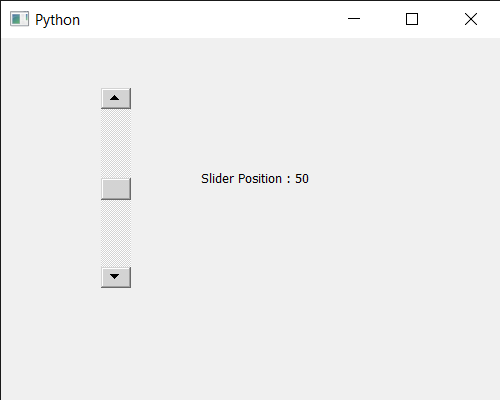GFG App
Open AppBrowser
Continue

# PyQt5 QScrollBar – Getting Slider Position

In this article we will see how we can get position of slider in QScrollBar. QScrollBar is a control that enables the user to access parts of a document that is larger than the widget used to display it. Slider is the scroll-able object inside the bar. We can change its position with the help of mouse and keyboard. Also we can change its position programmatically with the help of setSliderPosition method.

In order to do this we will use sliderPosition method with the scroll bar object.
Syntax : scroll.sliderPosition()
Argument : It takes no argument
Return : It returns integer

Example: Below is the implementation

## Python3

 `# importing libraries` `from` `PyQt5.QtWidgets ``import` `*` `from` `PyQt5 ``import` `QtCore, QtGui` `from` `PyQt5.QtGui ``import` `*` `from` `PyQt5.QtCore ``import` `*` `import` `sys`     `class` `Window(QMainWindow):`   `    ``def` `__init__(``self``):` `        ``super``().__init__()`   `        ``# setting title` `        ``self``.setWindowTitle(``"Python "``)`   `        ``# setting geometry` `        ``self``.setGeometry(``100``, ``100``, ``500``, ``400``)`   `        ``# calling method` `        ``self``.UiComponents()`   `        ``# showing all the widgets` `        ``self``.show()`       `    ``# method for components` `    ``def` `UiComponents(``self``):`   `        ``scroll ``=` `QScrollBar(``self``)`   `        ``# setting geometry of the scroll bar` `        ``scroll.setGeometry(``100``, ``50``, ``30``, ``200``)`   `        ``# making its background color to green` `        ``scroll.setStyleSheet(``"background : lightgrey;"``)`   `        ``# setting slider position` `        ``scroll.setSliderPosition(``50``)`     `        ``# creating a label` `        ``label ``=` `QLabel(``"GeeksforGeeks"``, ``self``)`   `        ``# setting geometry to the label` `        ``label.setGeometry(``200``, ``100``, ``300``, ``80``)`   `        ``# making label multi line` `        ``label.setWordWrap(``True``)`   `        ``# getting current position of the slider` `        ``value ``=` `scroll.sliderPosition()`   `        ``# setting text to the label` `        ``label.setText(``"Slider Position : "` `+` `str``(value))`           `# create pyqt5 app` `App ``=` `QApplication(sys.argv)`   `# create the instance of our Window` `window ``=` `Window()`   `# start the app` `sys.exit(App.``exec``())`

Output :My Personal Notes arrow_drop_up